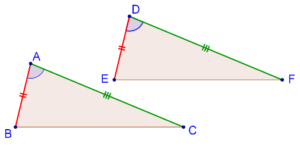# The SAS Criterion

Go back to  'Triangles-Quadrilaterals'

Now, we will start discussing the various conditions under which two triangles can be congruent.

The simplest criterion for the congruence of two triangles is the Side-Angle-Side criterion. This states that if two sides of one triangle, and the angle contained between these two sides, are respectively equal to two sides of another triangle and the angle contained between them, then the two triangles will be congruent. Let us understand this through a diagram.

Consider the following two triangles, ABC and DEF:Suppose that AB = DE, AC = DF. and ∠A = ∠D. Can you intuitively feel that the two triangles will be congruent? Suppose that you were to try and (exactly) superimpose ∆DEF.on ∆ABC. You would do this by moving the vertex D onto the vertex A., and aligning one side, say DE, with AB. Then, because ∠A = ∠D, the side DF will automatically become exactly aligned with the side AC, and so E would be lying on top of B, and F would be lying on top of C. This means that DF  will also be exactly aligned with and equal to BC.

Thus, you would be able to exactly superimpose ∆DEF on ∆ABC – the two triangles are thus congruent.

The SAS criterion is taken to be an axiom.